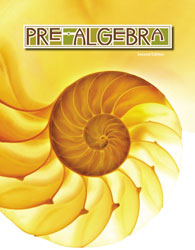## BJU Pre-Algebra Student Text (2nd Ed.)

• ₱2,410.00

Algebraic expressions and linear equations are applied throughout a thorough review of operations on integers, fractions, decimals, percents, and radicals. Students explore relations and functions using equations, tables, and graphs. Chapters on statistics and geometry extend foundational concepts in preparation for high school courses.

Highlights:

• Dominion Through Math exercises illustrate how mathematics can be used to steward God's creation for His glory.
• Problem Solving questions in each chapter promote critical-thinking skills and introduce the students to different problem-solving strategies.
• Math-in-Use studies feature Bible characters, mathematicians, scientists, and artists and highlight the math the person used.
• Math and Scripture features in each chapter highlight mathematical applications in God's Word.
• A Chapter Review at the end of each chapter includes a vocabulary review and some exercises.
• An expanded cumulative review is included in each section.
• Expanded explanations with numerous examples are included.
• Skill Check questions provide immediate feedback.
• Extended problem sets of three difficulty levels (A, B, and C) allow for customized assignments.
• Edition - 2nd ed.
• Item Code - 517060

We Also Recommend